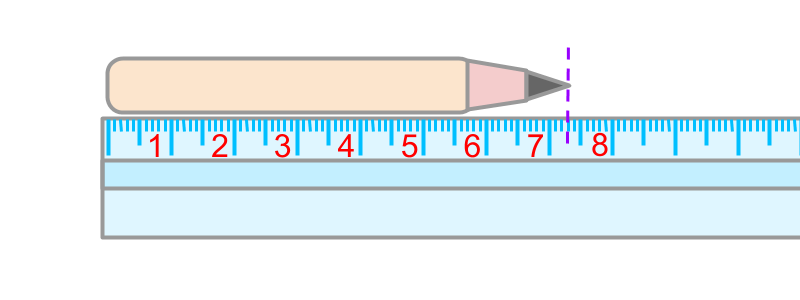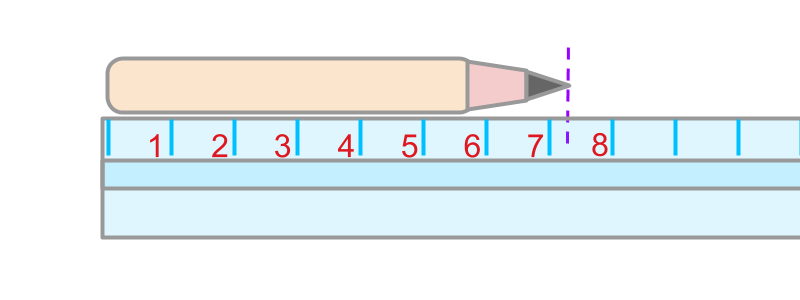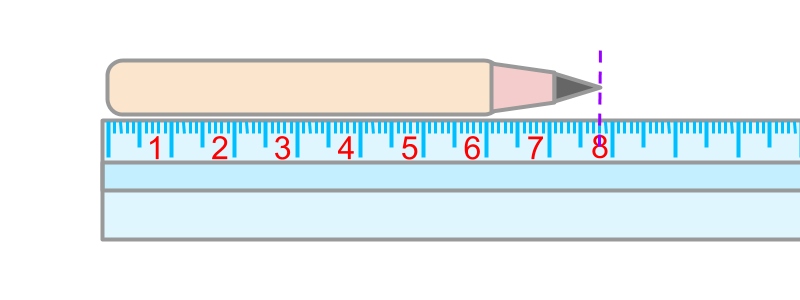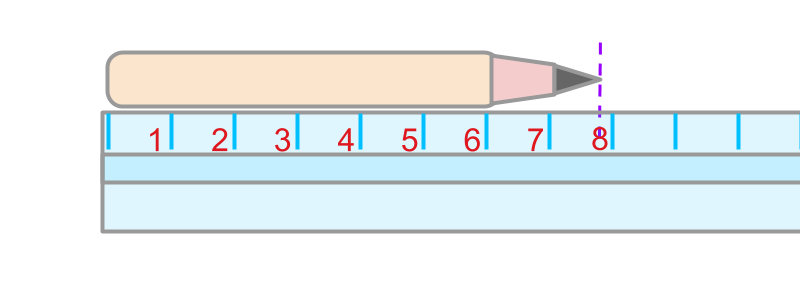maths > wholenumbers

Approximation and Estimation

what you'll learn...

overview

In this page, approximation of a number to the nearest "degree of approximation" is explained with a practical example.

In many applications, the value of measurement or count need not be accurate. It can be approximated or estimated to the nearest value to some degree.

Accurate and Approximate

The word "accurate" means: correct in details; exact.

The word "approximate" means: close to the actual; very near in value.The accurate length of the pencil in the figure is 73$73$mm.Let us consider a scale that does not have graduated marks for millimeters. Using the scale, the accurate length cannot be measured. The approximate length of the pencil in the figure is 7$7$ centimeter. Note that the value 7$7$ is closer to the accurate length.

EstimationThe accurate length of the pencil in the figure is 78$78$mm.Considering the scale with only centimeter marks, the approximate length of the pencil is measured as 8$8$ centimeter. Note that the value 8$8$ is closer to the accurate length.

In many applications, the value of measurement or count need not be accurate. It can be approximated to the nearest value to some degree.

The degree of approximation is explained as follows

•  $3842$ approximated to nearest $10$ is $3840$. The degree of approximation is to the nearest $10$.

•  $3842$ approximated to nearest $100$ is $3800$. The degree of approximation is to the nearest $100$.

•  $3842$ approximated to nearest $1000$ is $4000$. The degree of approximation is to the nearest $1000$.

The word "degree" means amount or level to which something is present. Degree of approximation is the level to which something is approximated.

The word "estimation" means: approximation and rough calculation to the nearest value. The estimation of a count or measure is the approximate value of the count or measure.

Approximation of numbers : A number may be approximated or rounded to the nearest $10$, or $100$, or $1000$, etc. If the number is halfway or above, then it is approximated to the higher value. If not, then it is approximated to the lower value.

Examples

The approximate value of $3428$ to the nearest $100$ is $3400$.

Estimate the value of $428$ to the nearest $10$ : The value of $428$ to the nearest 10 is $430$.

The approximate value of $3450$ to the nearest $100$ is $3500$.

Summary

Approximation of numbers : A number may be approximated or rounded to the nearest $10$, or $100$, or $1000$, etc. If the number is halfway or above, then it is approximated to the higher value. If not, then it is approximated to the lower value.

The degree of approximation is explained as follows

•  $3842$ approximated to nearest $10$ is $3840$. The degree of approximation is to the nearest $10$.

•  $3848$ approximated to nearest $10$ is $3850$. The degree of approximation is to the nearest $10$.

•  $3842$ approximated to nearest $100$ is $3800$. The degree of approximation is to the nearest $100$.

•  $3842$ approximated to nearest $1000$ is $4000$. The degree of approximation is to the nearest $1000$.

Outline